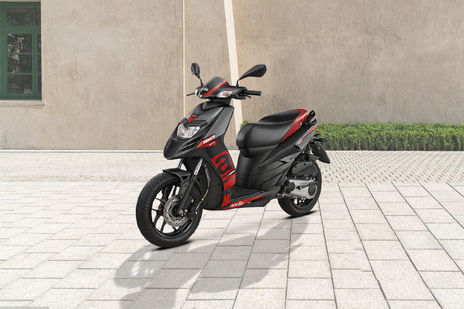# Aprilia SR 150 EMI Calculator50 reviews
Rs. 77,360 - 1.07 Lakh*
in delhi

Aprilia SR 150 EMI starts at Rs 2,972 per month for a tenure of 36 months @9.7 for a loan amount of Rs 82,698. The EMI Calculator tool on BikeDekho gives a detailed break-up of the total payable amount and helps you in finding the best bike finance for your SR 150. (Calculated on On Road Price in Delhi)

## Aprilia SR 150 Down Payment and EMI

SR 150 VariantsLoan @ 9.7%. Down paymentEMI (36 months)
Carbon Connectivity ABS90,954Rs. 10,106Rs. 3,266
Carbon ABS1,14,412Rs. 12,712Rs. 4,093
ABS1,11,334Rs. 12,371Rs. 4,006
Carbon STD82,929Rs. 9,214Rs. 2,982
STD82,698Rs. 9,189Rs. 2,972

## Calculate your Loan EMI for SR 150

Down PaymentRs.
0Rs.0
Bank Interest Rate %
8%26%
Loan Period ( Months )
• Total Loan AmountRs.0
• Payable AmountRs.0
• You’ll pay extraRs.0
EMIper month
Rs0
Calculated on On Road Price in delhi

## Find EMI of SR 150 Alternatives

*Ex-showroom Price in delhi

## Popular Bikes

•Yamaha YZF R15 V3
Rs.1.40 - 1.42 Lakh*
•Royal Enfield Classic 350
Rs.1.45 - 1.81 Lakh*
•Hero Splendor Plus
Rs.52,800 - 63,110*
•Honda Activa 5G
Rs.55,934 - 58,199*
•KTM 200 Duke
Rs.1.62 - 1.72 Lakh*
*Ex-showroom price in Delhi

## SR 150 Running Cost

KM driven per day20 km/day
Monthly fuel costRs.0* / month

## Frequently Asked Questions on Aprilia SR 150 EMI

### What will be the lowest down payment for Aprilia SR 150?

The lenders generally finance 90% of the value of the . Some customers might be eligible for 100% funding too. The down payment is the difference between the on-road price of the and the amount funded by a lender. This means the minimum possible down payment that you have to pay for Aprilia SR 150 includes the RTO and insurance charges.

### What will be the monthly EMI for Aprilia SR 150?

EMIs or Equated Monthly Installments refer to the monthly payments you make to the lender to repay your loan. These payments include the principal amount as well as the interest i.e. EMI = Principal Amount + Interest on Principal amount. Mathematically, EMI for Aprilia SR 150 can be calculated using the following formula: {P x R x (1+R)^N / [(1+R)^N-1]} where, P = Principal amount of the loan, R = Rate of interest and N = Number of monthly installments. For Example:- If the principal amount for a bike loan is Rs. ₹82,887/- on an annual rate of interest of 10% for a tenure of 3 years then  EMI = 82887 * 0.008333*(1+ 0.008333)^36 / ((1+ 0.008333)^36)-1 = Rs 2,972/- The rate of interest (R) on your loan is calculated monthly i.e. (R= Annual rate of interest/12/100). For instance, if R = 10% per annum, then R= 10%/12 = 0.008333.

### What will be the interest rate for Aprilia SR 150?

The interest rate for Aprilia SR 150 primarily depends on the principal amount and tenure of the loan amount. Interest rate of lenders generally varies from 9.7% per annum to 15% per annum. Customers can also negotiate with the financier for a better rate of interest against their loan amount.

### What will be the EMI for 3 years tenure for Aprilia SR 150?

EMI for Aprilia SR 150 for 3 years tenure can be calculated as per the following formula:- {P x R x (1+R)^N / [(1+R)^N-1]} Where, P = Principal amount of the loan, R = Rate of interest, N = Number of monthly installments which will be 36 in case of 3 years tenure.

Disclaimer :

Disclaimer : As per the information entered by you the calculation is performed by EMI Calculator and the amount of installments does not includes any other fees charged by the financial institution / banks like processing fee, file charges, etc. The amount is in Indian Rupee rounded off to the nearest Rupee. Depending upon type and use of vehicle, regional lender requirements and the strength of your credit, actual down payment and resulting monthly payments may vary. Exact monthly installments can be found out from the financial institution.

×